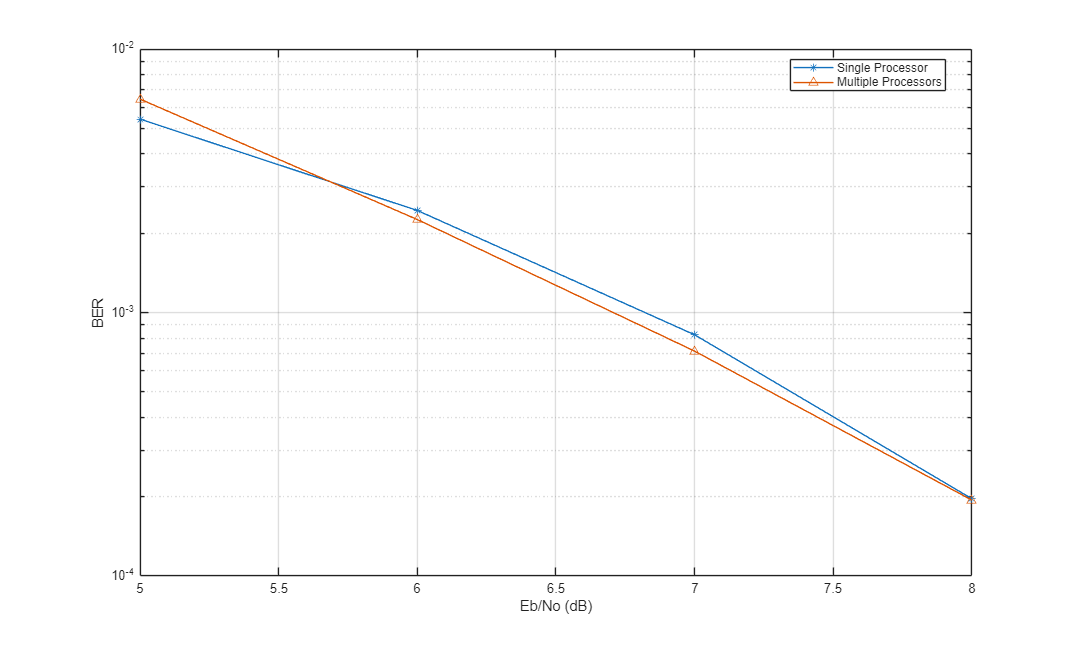# Accelerating BER Simulations Using the Parallel Computing Toolbox

This example uses Parallel Computing Toolbox to accelerate a simple, QPSK bit error rate (BER) simulation. The system consists of a QPSK modulator, a QPSK demodulator, an AWGN channel, and a bit error rate counter. In this example, four parallel processors are used.

Set the simulation parameters.

```EbNoVec = 5:8; % Eb/No values in dB totalErrors = 200; % Number of bit errors needed for each Eb/No value totalBits = 1e7; % Total number of bits transmitted for each Eb/No value ```

Allocate memory to the arrays used to store the data generated by the function, `helper_qpsk_sim_with_awgn`.

```[numErrors, numBits] = deal(zeros(length(EbNoVec),1)); ```

Run the simulation and determine the execution time. Only one processor will be used to determine baseline performance. Accordingly, observe that the normal for-loop is employed.

```tic for idx = 1:length(EbNoVec) errorStats = helper_qpsk_sim_with_awgn(EbNoVec, idx, ... totalErrors, totalBits); numErrors(idx) = errorStats(idx,2); numBits(idx) = errorStats(idx,3); end simBaselineTime = toc; ```

Calculate the BER.

```ber1 = numErrors ./ numBits; ```

Rerun the simulation for the case in which Parallel Computing Toolbox is available. Create a pool of workers.

```pool = gcp; ```
```Starting parallel pool (parpool) using the 'local' profile ... Connected to the parallel pool (number of workers: 6). ```

Determine the number of available workers from the `NumWorkers` property of `pool`. The simulation runs the range ofvalues over each worker rather than assigning a singlepoint to each worker as the former method provides the biggest performance improvement.

```numWorkers = pool.NumWorkers; ```

Determine the length of `EbNoVec` for use in the nested `parfor` loop. For proper variable classification, the range of a for-loop nested in a `parfor` must be defined by constant numbers or variables.

```lenEbNoVec = length(EbNoVec); ```

Allocate memory to the arrays used to store the data generated by the function, `helper_qpsk_sim_with_awgn`.

```[numErrors, numBits] = deal(zeros(length(EbNoVec),numWorkers)); ```

Run the simulation and determine the execution time.

```tic parfor n = 1:numWorkers for idx = 1:lenEbNoVec errorStats = helper_qpsk_sim_with_awgn(EbNoVec, idx, ... totalErrors/numWorkers, totalBits/numWorkers); numErrors(idx,n) = errorStats(idx,2); numBits(idx,n) = errorStats(idx,3); end end simParallelTime = toc; ```

Calculate the BER. In this case, the results from multiple processors must be combined to generate the aggregate BER.

```ber2 = sum(numErrors,2) ./ sum(numBits,2); ```

Compare the BER values to verify that the same results are obtained independent of the number of workers.

```semilogy(EbNoVec',ber1,'-*',EbNoVec',ber2,'-^') legend('Single Processor','Multiple Processors','location','best') xlabel('Eb/No (dB)') ylabel('BER') grid ```You can see that the BER curves are essentially the same with any variance being due to differing random number seeds.

Compare the execution times for each method.

```fprintf(['\nSimulation time = %4.1f sec for one worker\n', ... 'Simulation time = %4.1f sec for multiple workers\n'], ... simBaselineTime, simParallelTime) ```
```Simulation time = 67.2 sec for one worker Simulation time = 17.9 sec for multiple workers ```

In this case where four processor cores were used, the speed improvement factor was approximately four.